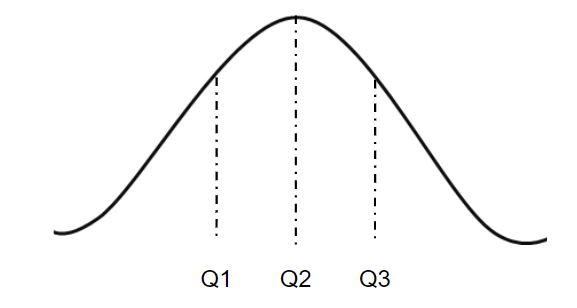# numpy.quantile() in Python

`numpy.quantile(arr, q, axis = None)` : Compute the qth quantile of the given data (array elements) along the specified axis.

Quantile plays a very important role in Statistics when one deals with the Normal Distribution.In the figure given above, `Q2` is the `median` of the normally distributed data. `Q3 - Q2` represents the Interquantile Range of the given dataset.

Parameters :
arr : [array_like]input array.
q : quantile value.
axis : [int or tuples of int]axis along which we want to calculate the quantile value. Otherwise, it will consider arr to be flattened(works on all the axis). axis = 0 means along the column and axis = 1 means working along the row.
out : [ndarray, optional]Different array in which we want to place the result. The array must have same dimensions as expected output.

Results : qth quantile of the array (a scalar value if axis is none) or array with quantile values along specified axis.

Code #1:

 `# Python Program illustrating  ` `# numpy.quantile() method  ` `import` `numpy as np ` ` `  ` `  `# 1D array  ` `arr ``=` `[``20``, ``2``, ``7``, ``1``, ``34``] ` ` `  `print``(``"arr : "``, arr)  ` `print``(``"Q2 quantile of arr : "``, np.quantile(arr, .``50``)) ` `print``(``"Q1 quantile of arr : "``, np.quantile(arr, .``25``)) ` `print``(``"Q3 quantile of arr : "``, np.quantile(arr, .``75``)) ` `print``(``"100th quantile of arr : "``, np.quantile(arr, .``1``))  ` `   `

Output :

```arr : [20, 2, 7, 1, 34]
Q2 quantile of arr : 7.0)
Q1 quantile of arr : 2.0)
Q3 quantile of arr : 20.0)
100th quantile of arr : 1.4)
```

Code #2:

 `# Python Program illustrating  ` `# numpy.quantile() method  ` `import` `numpy as np ` `  `  `# 2D array  ` `arr ``=` `[[``14``, ``17``, ``12``, ``33``, ``44``],   ` `       ``[``15``, ``6``, ``27``, ``8``, ``19``],  ` `       ``[``23``, ``2``, ``54``, ``1``, ``4``, ]]  ` `print``(``"\narr : \n"``, arr)  ` `    `  `# quantile of the flattened array  ` `print``(``"\n50th quantile of arr, axis = None : "``, np.quantile(arr, .``50``))  ` `print``(``"0th quantile of arr, axis = None : "``, np.quantile(arr, ``0``))  ` `    `  `# quantile along the axis = 0  ` `print``(``"\n50th quantile of arr, axis = 0 : "``, np.quantile(arr, .``25``, axis ``=` `0``))  ` `print``(``"0th quantile of arr, axis = 0 : "``, np.quantile(arr, ``0``, axis ``=` `0``))  ` `   `  `# quantile along the axis = 1  ` `print``(``"\n50th quantile of arr, axis = 1 : "``, np.quantile(arr, .``50``, axis ``=` `1``))  ` `print``(``"0th quantile of arr, axis = 1 : "``, np.quantile(arr, ``0``, axis ``=` `1``))  ` `  `  `print``(``"\n0th quantile of arr, axis = 1 : \n"``,  ` `   ``np.quantile(arr, .``50``, axis ``=` `1``, keepdims ``=` `True``)) ` `print``(``"\n0th quantile of arr, axis = 1 : \n"``,  ` `   ``np.quantile(arr, ``0``, axis ``=` `1``, keepdims ``=` `True``)) `

Output :

```arr :
[[14, 17, 12, 33, 44], [15, 6, 27, 8, 19], [23, 2, 54, 1, 4]]

50th quantile of arr, axis = None : 15.0
0th quantile of arr, axis = None : 1)

50th quantile of arr, axis = 0 : [14.5  4.  19.5  4.5 11.5]
0th quantile of arr, axis = 0 : [14  2 12  1  4]

50th quantile of arr, axis = 1 : [17. 15.  4.]
0th quantile of arr, axis = 1 : [12  6  1]

0th quantile of arr, axis = 1 :
[[17.]
[15.]
[ 4.]]

0th quantile of arr, axis = 1 :
[
[ 6]
[ 1]]
```

My Personal Notes arrow_drop_upAspire to Inspire before I expire

If you like GeeksforGeeks and would like to contribute, you can also write an article using contribute.geeksforgeeks.org or mail your article to contribute@geeksforgeeks.org. See your article appearing on the GeeksforGeeks main page and help other Geeks.

Please Improve this article if you find anything incorrect by clicking on the "Improve Article" button below.

Article Tags :

Be the First to upvote.

Please write to us at contribute@geeksforgeeks.org to report any issue with the above content.